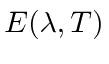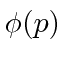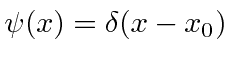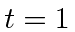## HOMEWORK 1

1. A polished Aluminum plate is hit by beams of photons of known energy. It is measured that the maximum electron energy iseV for 2000 Angstrom light andeV for 2580 Angstrom light. Determine Planck's constant and its error based on these measurements.

2. A 200 keV photon collides with an electron initially at rest. The photon is observed to scatter at 90 degrees in the electron rest frame. What are the kinetic energies of the electron and photon after the scattering?

3. Use the energy density in a cavity as a function of frequency and Tto calculate the emissive power of a black bodyas a function of wavelength and temperature.

4. What is the DeBroglie wavelength for each of the following particles? The energies given are the kinetic energies.
• a 1 eV electron
• aMeV proton
• a 1 gram lead ball moving with a velocity of 100 cm/sec.

5. The Dirac delta function has the property thatFind the momentum space wave functionif.

6. Use the calculation of a spreading Gaussian wave packet to find the fractional change in size of a wave packet betweenandsecond for an electron localized to 1 Angstrom. Now find the fraction change for a 1 gram weight localized to 1 nanometer.

7. Use the uncertainty principle to estimate the energy of the ground state of a harmonic oscillator with the Hamiltonian.

8. Estimate the kinetic energy of an electron confined to be inside a nucleus of radius 5 Fermis. Estimate the kinetic energy of a neutron confined inside the same nucleus.

Jim Branson 2013-04-22